##### Actions

convexity limit,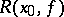, of a functionThe least upper bound of the radiiof the spheres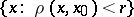, each one of which is mapped into a convex domain; here, the functionis defined on a domainof a metric space with metric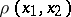and assumes values in a linear space. The convexity radius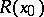at a point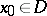with respect to some class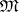of mappingsof the domainis, by definition, the number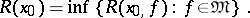Ifis an affine mapping of a Euclidean space,, then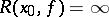. With respect to the classof all normalized univalent conformal mappings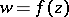,,, of the unit discof the complex plane, the convexity radius equals; if one imposes the additional condition of convexity of the domains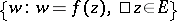, i.e. for convex functions (cf. Convex function (of a complex variable)),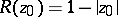. See also Univalent function.